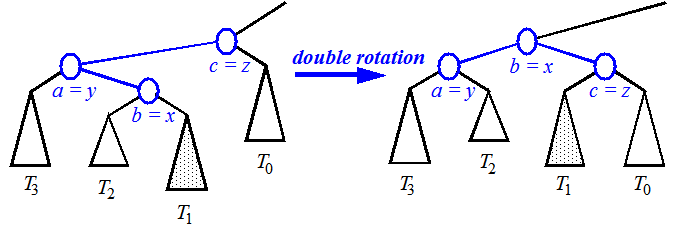磨刀不误砍柴工 系列之 一 : Heap BST AVL

(磨刀不误砍柴工) 系列是我学习算法的笔记，总结自己学习中的理解，并希望能分享交流。

PS: 虽然看算法书有点枯燥，但是这是学习算法的必经之路。

• Algorithm Design(Foundations, Analysis, and Intent Examples)</li>
• 算法导论(第3版)

Heap

Previously, I thought Heap is built in O(nlogn) time.
But sometimes, it can also be constructed in O(n) time.

PriorityQueue is NOT necessarily a Heap. Heap is a good implementation of PriorityQueue.

Bottom-Up Heap Construction runs in O(n) time.

Here is the pseudo-code of this construction method, it’s a recursive algorithm.

Algorithm BottomUpHeap($$S$$):
Input: A sequence $$S$$ storing $$n = 2^h - 1$$ keys
Output: A heap $$T$$ stroing the keys in $$S$$.
if S is empty then
return an empty heap (consisting of a single external node).
Remove the first key, $$k$$, from $$S$$.
Split $$S$$ into two sequences, $$S_1$$ and $$S_2$$, each of size $$(n-1)/2$$.
$$T_1$$ <– BottomUpHeap((S_1))
$$T_2$$ <– BottomUpHeap((S_2))
Create binary tree $$T$$ with root $$r$$ storing $$k$$, left subtree $$T_1$$, and right subtree $$T_2$$.
Perform a down-heap bubbling from the root $$r$$ of $$T$$, if necessary.
return $$T$$

BST

I have implemented the algorithm of a BST without the RemoveElement method before. And the RemoveElement is the most complicated among those common methods of BST.

Here is a good code example of BST. But where I found a bug in remove method. In Node with 2 children scenario, after we copied the data from the leftMost_node of right_subTree(we call it replace_node) to replace the deleted node, we should preserve the replace_node's right_subTree. This means, the replace_node should not be straightly deleted, we need to link its parent’s *left_child to its right_subTree beforehand.

void BinarySearchTree::remove(int d)
{
...
//Node with 2 children
// replace node with smallest value in right subtree
if (curr->left != NULL && curr->right != NULL) {
...
if((curr->right)->left != NULL) {
...
while(lcurr->left != NULL)
{
lcurrp = lcurr;
lcurr = lcurr->left;
}
curr->data = lcurr->data;
lcurrp->left = lcurr->right;
delete lcurr;
//lcurrp->left = NULL;
}
...
}

#16 is what I have fixed to replace #18.

BST VS. Heap

I was used to mix BST and Heap up. Now I know they are both elementary trees, but totally different.
BST is more well organized where every node satisfies left_subtree < node < right_subtree;
Heap does not necessarily so. What’s more, Heap is a complete tree.

eg: In a MinHeap, root is the minimum of the whole tree. An new node is inserted at the last position at first, then rejust its final position through Up-Heap Bubbling.

While in BST, a new node is compared from ROOT to its real position before it is inserted at the real position. And the real position of a new node must be a leaf.

AVL

A type of restructured BST to be balanced, named after the initials of its inventors: Adel’son-Vel’skii and Landis.

Height-Balance Property: For every internal node v of T, the heights of the children of v can differ by at most 1.

The height of an AVL tree storing n keys is O(lohn).

The insertion and removal operations for AVL trees are similar to those for binary search trees, but with AVL trees we must perform additional computations.

Insertion

An insertion in an AVL tree T begins as in an insertItem operation in BST.

While doing restructure on T, we maintaining the inorder relationships of all the nodes in T.

The modification of a tree T caused by a trinode restructure operation is ofen call a rotation, because of the geometric way we can visualize the way it changes T. (Ranger said: I have never figured out that this operation has any similarity as roatation visually. Just kidding.)

single roatation & double rotation

If b = y, the trinode restructure mothod is called a single rotation; Otherwise, if b = x, the trinode restructure operation is called a double rotation.signle roation Right-Rightsignle roation Left-Leftsignle roation Right-Leftsignle roation Left-Right

• One rotation (single or double) is sufficient to restore the height-balance in an AVL tree after an insertion.

Removal

We also use trinode restructuring to restore balance in the tree T.

In particular, let $$w$$ be the parent of the previously removed node. Let $$z$$ be the first unbalanced node encoundtered going up from $$w$$ toward the root of T. Also, let ye be the child of $$z$$ with larger height (note that node $$y$$ is the child of $$z$$ that is not an ancestor of $$w$$), and let $$x$$ be a child of $$y$$ with larger height.

• A single trinode restructuring may not restore the height-balance property globally after a removal. O(logn) trinode restructurings are sufficient.

Running Times for AVL Trees

• a single restructure is $$O(1)$$
• find is $$O(log n)$$
height of tree is $$O(log n)$$, no restructures needed
• insert is $$O(log n)$$
initial find is $$O(log n)$$
Restructuring up the tree, maintaining heights is $$O(log n)$$
• remove is $$O(log n)$$
initial find is $$O(log n)$$
Restructuring up the tree, maintaining heights is $$O(log n)$$

There are some points confused me in AVL:

• How does AVL detect the diff between height of left_child and right_right IN DETAIL?
• What’s the algorithm details of rotate operation? Will the 4-types rotation be very complicated?
 Tweet